# Pouring alcohol

100 liters of alcohol has 70%
How many liters of water need to be added to have 60% alcohol?

Correct result:

x =  16.667

#### Solution:

100 • 0.70 = 0.6 • (100+x)

0.6x = 10

x = 503 ≈ 16.666667

Calculated by our simple equation calculator.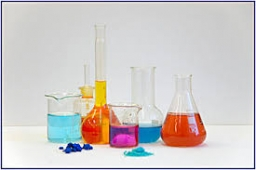Our examples were largely sent or created by pupils and students themselves. Therefore, we would be pleased if you could send us any errors you found, spelling mistakes, or rephasing the example. Thank you!

Leave us a comment of this math problem and its solution (i.e. if it is still somewhat unclear...):Be the first to comment!Tips to related online calculators
Do you have a linear equation or system of equations and looking for its solution? Or do you have quadratic equation?
Tip: Our volume units converter will help you with the conversion of volume units.

## Next similar math problems:

• The Chemistry testThe Chemistry test contained 8 questions, each with 3 points. Peter scored 21 points. How many percent did Peter write a test?
• Art schoolEvery fifth pupil 9A goes to art school. How many percent of pupils in class 9A go to art school?
• PercentCalculate how many % is the number 26.25 less than the number 105.
• Profit gainIf 5% more is gained by selling an article for Rs. 350 than by selling it for Rs. 340, the cost of the article is:
• Ice skates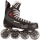Ice skates were raised twice, the first time by 25%, the second time by 10%. After the second price, their cost was 82.5 euros. What was the original price of skates?
• Percentages52 is what percent of 93?
• PupilsThere are 350 girls in the school, and the other 30% of the total number of pupils are boys. How many pupils does the school have?
• Cinema ticketsCinema sold 180 tickets this Thursday, which is 20%. Monday 14%, Tuesday 6%, Wednesday 9%, Friday 24%, Saturday 12%, and Sunday 15%. How many tickets were sold per week?
• GirlsThe children's competition was attended by 63 girls, which is 30% of all children's participants. How many children attended this competition?
• SummerjobThe temporary workers planted new trees. Of the total number of 500 seedlings, they managed to plant 426. How many percents did they meet the daily planting limit?
• Copper sulphate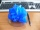How much g of water do we have to add to 240 g of an 84% CuSO4 solution to produce a 60% solution? (Express the mass of crystalline CuSO4 in the original solution and in the resulting solution and compare them. )
• Reducing numberReducing the an unknown number by 28.5% we get number 243.1. Determine unknown number.
• Perctentages35% of what number is 35?
• Unknown number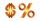15% of the unknown number is 18 less than 21% of the same number. What is the unknown number?
• Sale offThe TV went down 10% and then 10% off the original price again. Now it costs 300 €. What was its original price?
• Reward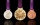Janko and Peter divided the reward from the brigade so that Peter got 5/8 of the reward. What percentage of Janko's reward got?
• Fifth of the numberThe fifth of the number is by 24 less than that number. What is the number?